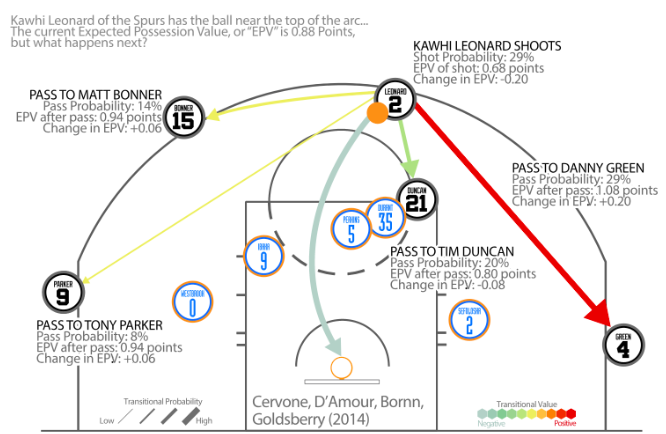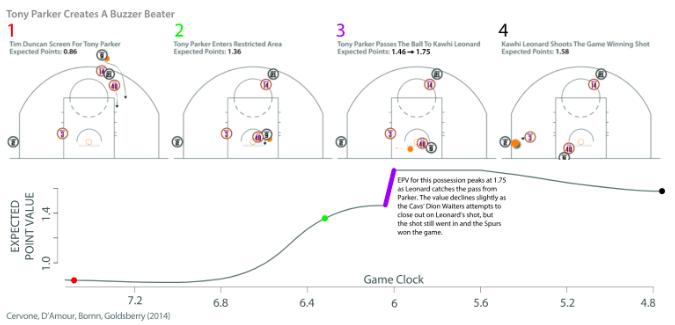# The SolutionDiagram of EPV as a weight average of the values of the ballcarrier’s decisions and the probability of making each decision.

# EPV (Expected Possession Value)

`EPV = E[points | d(t)]`EPV throughout the Spurs’ final possession, with annotations of major events.

# Introducing EPVmetrics

`Shot Satisfaction = Σ (EPV(t) - E[points | pass in (t, t+ε], d(t)])`

--

--